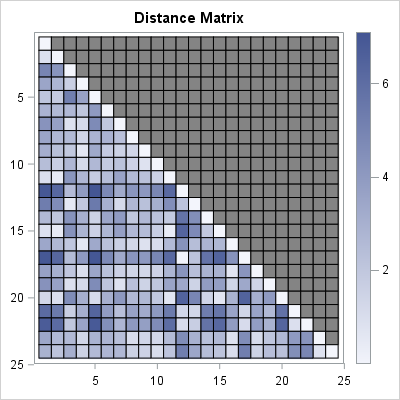Have you ever noticed that some SAS/IML programmers use the CALL statement to call a subroutine, whereas others use the RUN statement? Have you ever wondered why the SAS/IML language has two statements that do the same thing?

It turns out that the CALL statement and the RUN statement do not do the same thing! Read on to discover how they differ.

By the way, the RUN statement in PROC IML has only one purpose: to execute a subroutine. Many other SAS procedures (such as REG, GLM, and DATASETS) use the RUN statement to tell SAS to run the procedure. PROC IML is different. Never put the RUN statement at the end of a PROC IML program.

### Built-in versus user-defined subroutines

A main feature of the SAS/IML language is that it enables you to define your own modules. A module is a user-defined function or subroutine that you can call from an IML program. A module that returns a value is called a function module; a module that does not return a value is called a subroutine module.

Although I do not recommend that you do so, it is possible to define a subroutine module that has the same name as a built-in subroutine. These two routines co-exist. If you use the RUN statement, SAS/IML will call the subroutine that you wrote. If you use the CALL statement, SAS/IML will call the built-in subroutine.

So that's the difference between the RUN and CALL statements: The RUN statement looks first for a user-defined module with the specified name. If it finds it, then it calls that module. Otherwise, it calls the built-in subroutine that has the specified name. The CALL statement looks for a built-in subroutine and immediately calls that routine if it is found.

### An example: Overriding the EIGEN subroutine

As I said, I don't recommend that you routinely write user-defined modules that have the same name as a SAS/IML built-in, but let's examine a situation in which it might be convenient to do so. Let's assume that you often read data sets that represent symmetric matrices, and that these data sets are stored in lower triangular form with missing value in the upper triangular portion of the matrix. For example, this is the default storage method for distance matrices that are created by using the DISTANCE procedure in SAS/STAT software, as shown below:

```proc distance data=Sashelp.Cars out=Dist method=Euclid; where Type="Truck"; var interval(Horsepower--Length / std=Std); id Model; run;   proc iml; use Dist; read all var _NUM_ into D[r=Model]; close Dist; call heatmapcont(D) title="Distance Matrix";```The program creates a 24 x 24 symmetric matrix of distances between 24 observations in a six-dimensional space of variables. The program reads the distance matrix into a SAS/IML matrix. A heat map shows the matrix values. The dark gray color shows that the upper triangular portion of the matrix is missing. The white diagonal elements show that the diagonal of the matrix is exactly zero. The remaining cells indicate the distance between observations. Nearly white cells indicate that two observations are close together. Dark cells indicate that observations are relatively far apart.

Suppose that you want to compute the eigenvalues and eigenvectors of this matrix. The built-in EIGEN subroutine in SAS/IML can compute these quantities, but it expects a matrix that does not have any missing values. Therefore to compute the eigenvalues you should first extract the lower triangular elements of the matrix and copy them into the upper triangular portion of the matrix.

You could write a separate subroutine that only copies the lower triangular elements, but for this example I will write a subroutine that has the same name as the built-in EIGEN subroutine. The following statements define a module that inspects the upper triangular elements of a matrix. If the upper triangular elements are all missing, it replaces those missing values with the lower triangular elements. It then calls the built-in EIGEN function to compute the eigenvalues and eigenvectors:

```start StrictLowerTriangular(X); /* return lower triangular elements */ return( remove(vech(X), cusum(1 || (ncol(X):2))) ); finish;   /* define EIGEN module as a custom override of the built-in subroutine */ start Eigen(eval, evec, A); r = row(A); c = col(A); UpperIdx = loc(c>r); /* if upper triangular elements are missing, copy from lower */ if all(A[UpperIdx]=.) then A[UpperIdx] = StrictLowerTriangular(A); call eigen(eval, evec, A); /* CALL the built-in EIGEN subroutine */ finish;   run eigen(eval, evec, D); /* RUN the user-defined EIGEN subroutine */```

In summary, the CALL and RUN statements enable you to choose between a built-in subroutine and a custom subroutine that have the same name.

The situation is a little different for user-defined functions. Beginning with SAS/IML 13.1, you can define a function that has the same name as a built-in function, and the order of resolution for calling functions ensures that a user-defined function is found before a built-in function that has the same name. This means that if you choose to override a SAS/IML function, you lose the ability to call the built-in function until you quit PROC IML.

Have you ever had the need to override a built-in SAS/IML subroutine? Let me know the details by leaving a comment.

Share1.